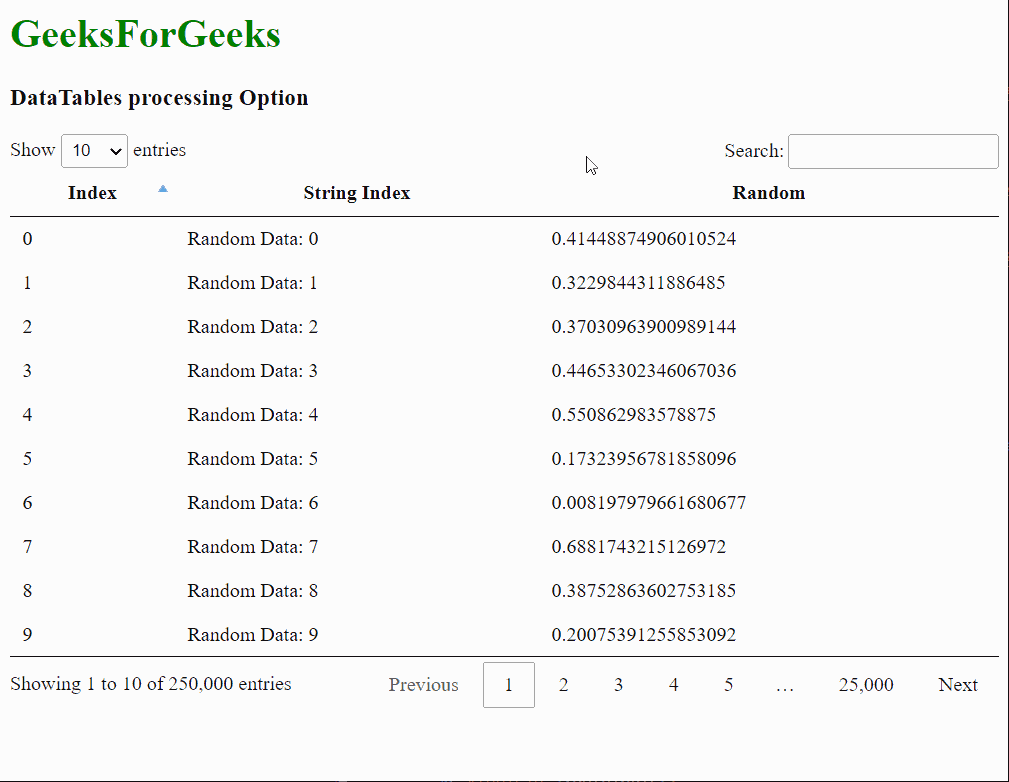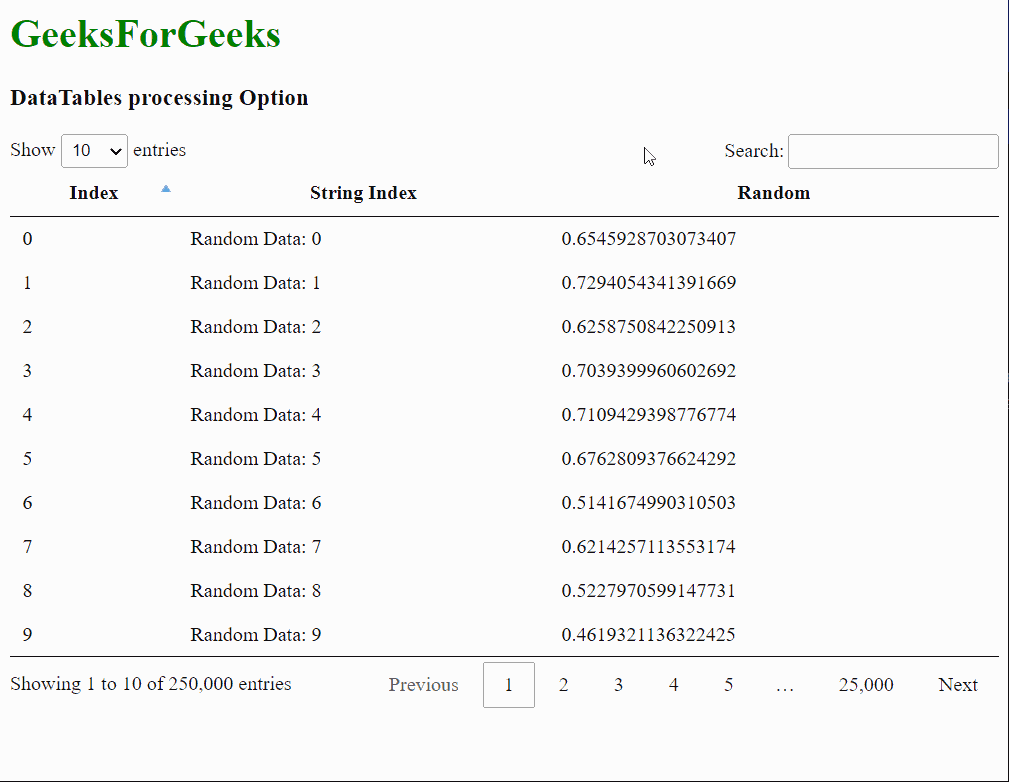GeeksforGeeks App
Open AppBrowser
Continue

## Related Articles

DataTables is jQuery plugin that can be used for adding interactive and advanced controls to HTML tables for the webpage. This also allows the data in the table to be searched, sorted, and filtered according to the needs of the user. The DataTable also exposes a powerful API that can be further used to modify how the data is displayed.

The processing option is used to specify whether the processing indicator is enabled or not. This indicator is shown when the DataTable is busy doing some operation that would take some time. This is useful for tables that have a huge amount of data and take a lot of time to perform any operation. This indicator would be able to notify the user that an operation is going on and has not got stuck. A true value displays the indicator and a false value removes it.

Syntax:

`{ processing: value }`

Option Value: This option has a single value as mentioned above and described below:

• value: This is a boolean value that specifies the processing indicator is enabled or not. The default value is false.

The below example illustrates the use of this option.

Example 1: This example enables the processing indicator that can be seen while sorting the columns.

## HTML

 `<``html``>`` ` `<``head``>``    ````    ``<``script` `type``=``"text/javascript"` `    ``src``=``"https://code.jquery.com/jquery-3.5.1.js"``>``    ```` ` `    ````    ``<``link` `rel``=``"stylesheet"` `href``=``"https://cdn.datatables.net/1.10.23/css/jquery.dataTables.min.css"``>`` ` `    ````    ``<``script` `src``=``"https://cdn.datatables.net/1.10.23/js/jquery.dataTables.min.js"``>``    `````` ` `<``body``>``    ``<``h1` `style``=``"color: green;"``>``        ``GeeksForGeeks``    ````    ``<``h3``>DataTables processing Option`` ` `    ````    ``<``table` `id``=``"tableID"` `class``=``"display"` `        ``style``=``"width: 100%;"``>``    ```` ` `    ``<``script``>`` ` `        ``// Initialize a huge dataset to ``        ``// see the effects of processing``        ``let dataset = [];``        ``for (let i = 0; i < ``250000``; i++) {``            ``let newArr =``                ``[i, "Random Data: " + i, Math.random()];``            ``dataset.push(newArr);``        ``}`` ` `        ``// Initialize the DataTable``        ``\$(document).ready(function () {``            ``\$('#tableID').DataTable({`` ` `                ``// Add the data created above``                ``data: dataset,``                ``columns: [``                    ``{ title: "Index" },``                    ``{ title: "String Index" },``                    ``{ title: "Random" },``                ``],`` ` `                ``// Enable the processing indicator``                ``// of the DataTable``                ``processing: true,``            ``});``        ``});``    `````` ` ``

Output:Example 2: This example disables the processing indicator.

## HTML

 `<``html``>`` ` `<``head``>``    ````    ``<``script` `type``=``"text/javascript"` `    ``src``=``"https://code.jquery.com/jquery-3.5.1.js"``>``    ```` ` `    ````    ``<``link` `rel``=``"stylesheet"` `href``=``"https://cdn.datatables.net/1.10.23/css/jquery.dataTables.min.css"``>`` ` `    ````    ``<``script` `src``=``"https://cdn.datatables.net/1.10.23/js/jquery.dataTables.min.js"``>``    `````` ` `<``body``>``    ``<``h1` `style``=``"color: green;"``>GeeksForGeeks``    ``<``h3``>DataTables processing Option`` ` `    ````    ``<``table` `id``=``"tableID"` `class``=``"display"``        ``style``=``"width: 100%;"``>``    ```` ` `    ``<``script``>`` ` `        ``// Initialize a huge dataset to ``        ``// see the effects of processing``        ``let dataset = [];``        ``for (let i = 0; i < ``250000``; i++) {``            ``let newArr =``                ``[i, "Random Data: " + i, Math.random()];``            ``dataset.push(newArr);``        ``}`` ` `        ``// Initialize the DataTable``        ``\$(document).ready(function () {``            ``\$('#tableID').DataTable({`` ` `                ``// Add the data created above``                ``data: dataset,``                ``columns: [``                    ``{ title: "Index" },``                    ``{ title: "String Index" },``                    ``{ title: "Random" },``                ``],`` ` `                ``// Disable the processing indicator``                ``// of the DataTable``                ``processing: false,``            ``});``        ``});``    `````` ` ``

Output:My Personal Notes arrow_drop_up
Related Tutorials